Module 1.3 Notes "Describing Data with Number Summaries"

 Index to Module One Notes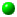1.1: Why Statistics for Mgt1.2: Describing Data: Pictures1.3: Describing Data: Number Summaries1.4: Estimating with Confidence1.5: Testing Hypothesis

Measures of Central Tendency

In describing a set of data, we are interested in the numerical measures of center and spread. Recall in the cycle time example of Module 1.2, the center of the data appears to be a cycle time of 21 days. That is formally called the measure of central tendency of the data. There are two classic ways to measure the center of a distribution of data: the mean and the median.

Mean
The mean or average is the arithmetic measure of central tendency, and is simply the sum of all of the observations in a set of data divided by the total number of observations. So, the mean of seven cycle times with values 21, 23, 19, 22, 20, 22, 20 is:

Mean = (21 + 23 + 19 + 22 + 20 + 22 + 20) / 7 = 21

You will read in statistics texts that this is the formula for both the population mean (its symbol is the Greek letter mu) and the sample mean (its symbol is an x with a bar over it, or x-bar). Don't get alarmed - this is not a course with a lot of formulas, symbols and computations - we will let the computer do it. I just want to highlight the computations for the classic measures in descriptive statistics. You will also read in textbooks that measures based on a population are called parameters, and measures based on a sample are called statistics.

Median
The median is called the location measure of central tendency since it is the middle value of an ordered array of observations - 50% of the observations in a set of data fall below the median provided the data is in an ordered array. Since we have to account for 100% of the data, 50% of the observations fall above the median. So, given the cycle times of 21, 23, 19, 22, 20, 22, 20; first created an ordered array (sorted list of the numbers):

Ordered array = 19, 20, 20 , 21, 22, 22, 23

The median is the ordered or ranked observation that appears at location = (n + 1)/2, where n is the symbol for the number of numbers in the set of data. For this ordered array:

Median = (7 + 1) / 2 = 4th ordered observation, which is the number 21.

Do you note that the mean and the median are equal? They will be approximately equal (such as within 5% of each other) as long as the distribution of the set of data is somewhat symmetric without extreme values (signals or outliers) to one side or the other. This is an important point. Suppose we had an outlier or signal cycle time of 38 instead of 23. Let's calculate the mean and the median:

Mean = (19, 20, 20, 21, 22, 22, 38) / 7 = 23.14

Median = (7 + 1) / 2 = 4th ordered observation, which is 21

The mean was "pulled" to the outlier value of 38 - the mean is not resistant to extreme values, but the median is since it is a location and not arithmetic measure. Note that the mean is about 10% greater than the median: 21 is more representative of the center when the distribution is not symmetric. As an aside, economists generally use the median when reporting the measure of center for housing values since sets of housing data are often skewed by high or low extreme values. It would be misleading or even unethical to report the mean housing value as a measure of center when there are extreme values on the high side - the median is more representative of center in that case. Also note that when the mean > median, the distribution is skewed right - you saw a histogram picture of that in Worksheet 1.2.3 of Module 1.2. Similarly, when the mean < median, the distribution would be skewed left - you saw a histogram picture of that in Worksheet 1.2.4 of Module 1.2.

Before we go on, let me show you how to compute the median with an even set of data. Suppose we have the numbers 19, 20, 20, 21, 22, 22, 23, and 23. The median is:

Median = (n + 1) / 2 = (8 + 1) / 2 = 4.5th ordered observation; the number at the 4.5th location is the average between the 4th and 5th numbers or 21.5

Mode
Sometimes you hear of the mode as a measure of central tendency. However, the mode is simply the most frequently appearing number in a data set, so there are actually two modes in the above distribution, 20 and 22. We will not be using the mode as a measure of central tendency, but only to help in general examination of shape, as discussed in Module 1.2.

Pause and Reflect

The mean and the median are measures of center. The mean and median will be approximately equal for symmetric sets of data. The median is resistant to extreme values, whereas the mean is pulled towards them. When the mean > median, the distribution is skewed right by an extreme value(s) > mean. When the mean < median, the distribution is skewed left by an extreme value(s) < mean. Thus, for sets of data with extreme values, the median is more representative of the center, and is the preferred measure. Alternatively, the extreme values could be removed from the data for separate study, and a new, more accurate arithmetic mean can then be computed.

Measures of Variation

Range
Equally important to describing the center of a set of data with a numerical measure, is describing the spread or variation in a set of data. There are three classic ways to measure variation. The simplest measure of spread is the range. In the data set, 19, 20, 20, 21, 22, 22, 23:

Range = Maximum Number - Minimum Number = 23 - 19 = 4

So there is a spread of four days between the smallest and largest cycle times.

Standard Deviation
The range is not very rich - it ignores all but two numbers. If the distribution is approximately normal or a symmetric bell-shape, the classic measure of variation is the standard deviation. To set the stage for the standard deviation, recall that the mean of these numbers is 21. The number 19 varies from 21 by - 2, the number 20 varies by -1, the number 23 by +2, and so forth. The standard deviation is simply a measure of the average of these deviations. To compute the standard deviation for a population of numbers 19, 20, 20, 21, 22, 22, 23; first find the mean (we already did that, it is 21). Now subtract each number from the mean, square the result (to get rid of plusses and minuses), sum the resulting squared deviations, and divide by the number of numbers. This is called the variance of the population. The standard deviation of the population (the symbol is the Greek letter sigma) is the square root of the variance:

Mean = (19 + 20 + 20 + 21 + 22 + 22 + 23) / 7 = 21

Sum of Squared Deviations = (19 - 21)2 + (20 - 21)2 + (20 - 21)2 + (21 - 21)2 + (22 - 21)2 + (22 - 21)2 + (23 - 21)2 = 4+1+1+0+1+1+4 = 12

Variance = 12 / 7 = 1.714

Standard Deviation = Square Root of 1.714 = 1.3

The standard deviation for a sample (the symbol is the letter s) is similar to the above, but the variance is computed by dividing the sum of squared deviations by the number of numbers minus one (n - 1). In Modules 1.4 and 1.5 we will adjust the sample size to n - 1 again, and refer to this as degrees of freedom. For large sample sizes, the impact of dividing by n - 1 versus dividing by n is negligible. For small samples, it makes a difference so dividing by n - 1 gives a larger or more conservative estimate of the spread. If the above set of data is a sample, the variance and standard deviation are:

Mean = 21
Sum of Squared Deviations = 12
Variance = 12 /(7 - 1) = 2
Standard Deviation = S = Square Root of 2 = 1.4

The standard deviation is interpreted with respect to the mean. For symmetric bell-shaped sets of data, the interpretation of the standard deviation is:

95% (most) of the observations fall between the mean + 2 Std Dev

This can be combined with areas incorporating 1 and 3 standard deviations to completely describe a set of approximately symmetric, bell-shaped data:

68% of the observations fall between the mean + 1 Std Dev

95% of the observations fall between the mean + 2 Std Dev

99.7% (almost all or 100%) of the observations fall between the mean + 3 Std Dev

This very important property is called the Empirical Rule - it applies to all symmetric bell shaped sets of data. We will apply it later when we get to a larger example.

Interquartile Range
The third measure of spread or variation is the interquartile range (IQR). To compute this measure, we need the 1st and 3rd quartiles (Q1 and Q3, respectively). Twenty five percent of the ordered observations in a set of data is contained within a quartile, so 25% of the observations are below the first quartile, 50% of the observations are below the 2nd quartile (Q2, also called the Median), and 75% of the observations are below the 3rd. The IQR is simple Q3 - Q1. Here are the computations, using the numbers 19, 20, 20, 21, 22, 22, 24.

Q1 = (n + 1)/4 ordered observation = 0.25 * (7 + 1) = 2nd ordered observation which is the first number 20.
where "*" is symbol for multiplication

Q3 = 3*(n + 1)/4 ordered observation = 0.75 * (7 + 1) = 6th ordered observation which is the second number 22 in the ordered array.

IQR = Q3 - Q1 = 22 - 20 = 2 days

The middle 50% of the data falls within the IQR. Sometimes, analysts like to expand the location measure of spread by reporting the five number summary:

Minimum, Q1, Median, Q3, Maximum

Pause and Reflect

The standard deviation and the interquartile range are measures of spread or variation. The standard deviation is associated with the mean and is an accurate measure of spread for symmetric bell-shaped distributions. The IQR is generally associated with the median, and is an accurate measure of spread for non-symmetric distributions. With two numerical measures (mean and standard deviation; or median and IQR) we gain much knowledge about a data set - even data sets of thousands of numbers.

Relative Measures

Symmetry
There are some quick numerical measures than can be used to supplement frequency distribution charts to determine if the distribution is symmetric, bell-shaped or skewed. They are:

Set of Data is skewed to left if mean < median
Set of Data is skewed to right if mean > median
Set of Data is symmetric, bell shaped if:
Mean is approximately equal to median
Empirical rule is met
IQR is approximately equal to 1.33 * Standard Deviation

We will try the above rules of thumb when we illustrate the Excel descriptive statistics with a larger sample later in this module.

Variation
The coefficient of variation is a useful tool when comparing the variation of one distribution to that of another, especially if the distributions have different units of measurement.

Coefficient of variation = (standard deviation / mean) * 100%
For the example: (1.4 / 21) * 100% = 6.67%

Suppose another company experiences mean cycle time of 21 in their supply chain as well, but the standard deviation is 7 days. The coefficient of variation in this case is 33%, which reflects a process with much greater variability than one with 6.67% coefficient of variation.

Observations
Relative measures of observations are useful to give observations context within a data set. The percentile is a relative measure of location for a number in an ordered array. We already discussed examples of percentiles, since the first quartile is actually the 25th percentile - 25% of the data in an ordered array are below the 25th percentile. Perhaps you recall your percentile score on college entrance exams. If you scored in the 90th percentile on the SAT, 90 percent of the ordered scores were below yours. To find the 90th percentile, order the observations, then compute 90th percentile = 0.90*(n+1) to find the ordered observation of interest. If n = 780, then we would be looking for the 703rd number.

For distributions that are approximately symmetric, bell-shaped, the Z-Score or Z- Value is a powerful relative measure for observations. The Z-Score is simply the number of standard deviations an observation is from the mean. So when we find the Z -Score of a number, we standardize that number. What is the Z-Score for the number 19.6 in a distribution with a mean of 21 and standard deviation of 1.4?

Z = (observation - mean)/standard deviation = (19.6 - 21)/1.4 = -1

We would say that the number 19.6 is 1 standard deviation from (to the left of) the mean of 21.

Z-Scores have associated probabilities. Do you recall that 68% of the observations in a symmetric bell-shaped distribution fall between the mean and + 1 standard deviation? That "1" standard deviation is the Z-Score! Note also the 68% - we can find the probability for getting any Z-Score as long as the set of data is normal or is approximately symmetric bell-shaped. For example, what is the probability of getting an observation less than 19.6 days? We know that theoretically, the range of data from 19.6 to 22.4 days includes 68% of the data, and since we have to have 100% of the data accounted for, 100% - 68% or 32% of the data must include numbers up to 19.6 and above 22.4. Since we assume these distributions are symmetric, 16% of the data is up to 19.6 and 16% is above 24. So, for a symmetric bell shaped distribution with mean of 21 and standard deviation of 1.4, the probability of observing a number less than 19.6 is 0.16 or 16%.; the probability of observing a number greater than 19.6 is simply 100% - 16% or 84%.

Using Excel for the Computations

We will use the Data Analysis Tool and Statistical Functions to produce the descriptive statistics we have discussed thus far in Module 1.3.

The quickest way to generate a family of descriptive statistics is to use the Data Analysis Descriptive Statistics Tool. Lets use the data from Worksheet 1.2.1 in Module 1.2. First create a column of the cycle times as shown in that Worksheet (or if you saved that example, copy C1:C31 to a new area of your worksheet past the histogram, such as Column J). I placed the numbers in column J, starting with the title "Time" in Row 1, and the 30 cycle times in J2 to J31. The data doesn't have to be sorted to compute the descriptive statistics, but it can be. Now select Tools from the Standard Toolbar, then Data Analysis from the pulldown menu, then select Descriptive Statistics and follow the dialog box requests as you did in creating the histogram. For output options, select Summary Statistics and Confidence Level for the Mean, leaving the confidence level default at 95%. I also like to put the output close to my data and histogram if I created a histogram for the data. Remember that you only have to enter one cell location for the Output Range, such as L1, and that cell will define the upper left-hand corner of the range of cells needed for the output - just be sure that the worksheet is clear below and to the right of the cell you select. Note that you can put your output on a separate worksheet or even in a separate workbook if you like. You should get Worksheet 1.3.1 below:

Worksheet 1.3.1

 Time Mean 21.07 Standard Error 0.54 Median 21 Mode 21 Standard Deviation 2.94 Sample Variance 8.62 Kurtosis 0.90 Skewness 0.89 Range 13 Minimum 16 Maximum 29 Sum 632 Count 30 Confidence Level(95.0%) 1.10

We have discussed the Mean, Median, Mode, Standard Deviation, Sample Variance, Range, Minimum, Maximum, Sum and Count (number of observations). You may ignore Kurtosis (a mathematical measure of the concentration of data around the center compared to the tails of the distribution) and Skewness (a mathematical measure of the symmetry of the distribution). You may ignore Standard Error and Confidence Level for now, but we will use them in Module 1.4

The Descriptive Statistics tool provides everything we have discussed then, except Q3 and Q1. To get these, we need to use the Function feature of Excel. As for all Excel Functions, position the cursor in a cell or select the cell in which you want the quartile, for example M31. Select Insert from the Standard Toolbar, then Function, then Statistical (in left box of Paste Function dialog screen), then Quartile (scroll down to find Quartile in right box of dialog screen), then follow the dialog screen by inserting the cell range for your data (e.g., L2:L30), and 1 for Q1. The resulting cell formula is =QUARTILE(L2:L30, 1). Note that no labels are allowed so I started the cell range with L2 rather than L1. You should get the results in Worksheet 1.3.2. I repeated this process for the third quartile, Q3, which I placed in M32. I also added the formula =(M32 - M31) in cell M33 to get the interquartile range. I added the labels in cells L31, L32, and L33 for clarity since the function feature does not insert the title of the function.

Worksheet 2

 First Quartile, Q1 = 19 Third Quartile, Q3 = 22 Interquartile Range = 3

Lets use the above descriptive statistics to completely describe the set of data.

Location Measures of Center and Spread
Fifty percent of the ordered observations fall below the median of 21 days. Twenty-five percent of the ordered observations fall below the first quartile of 19 days; and 75% of the ordered observations fall below the third quartile of 22 days. The interquartile range is 3 days from 19 to 22, representing the middle 50% of the ordered observations. The five number summary is complete when we add the minimum value of 16 and maximum of 29 days.

Arithmetic Measures of Center and Spread
The mean is 21.07, or simply 21 days depending on the accuracy we need for reporting purposes. The standard deviation is 2.94 or, rounded, 3 days. The interpretation: most or 95% of the observations fall within the interval: mean + 2 *s = 21 + (2 * 3) or 15 to 27 days. To use the Empirical Rule to completely summarize a set of data which is approximately symmetric and bell-shaped, and having a mean of 21 and standard deviation of 3:

68% of the observations are within 21 + (1 * 3): 18 to 24 days.

95% of the observations are within 21 + (2 * 3): 15 to 27 days.

100% of the observations are within 21 + (3 * 3): 12 to 30 days.

From the above, we would not expect any observations below 12 days or above 30. Such observations would be outliers.

Percentile
To find the percentile using Excel, select Insert on the Standard Toolbar, then Function, then Statistical, then Percentile, and then respond to the dialogue box by entering the cell range for your numbers and the desired percentile. For example, to get the 25th percentile for this illustration, the resulting cell formula will be: =PERCENTILE(L2:L31,0.25). The percentile will be placed in your spreadsheet wherever you have the cursor (active cell).

The Z-Score
What is the Z-Score for the number 18?

Z-Score = (number - mean)/standard deviation = (18 - 21)/3 = -1

So, 18 is one standard deviation to the left of the mean of 21. What is the Z-Score for the number 24?

Z-Score = (24 - 21)/3 = 1

The number 24 is one standard deviation to the right of the mean. To get the Z-Score from Excel, we use the Standardize function. Position the cursor or point and click in a cell in which you want to place the Z-Score, such as Q2. Select Insert from the Standard Toolbar, then Function, then Statistical, then Standardize and respond to the dialog box questions. You should replicate the cell formula =STANDARDIZE(18,21,3) which gives a Z-Score of -1. I actually prefer to use the cell address for the number 18 so that I can copy the standardize cell reference to standardize a whole row or column of numbers. This would be =STANDARDIZE(L6,21,3). I then add a title to the column, such as "Z-Scores." The fifth requirement in Project Assignment 1 is to standardize (generate Z-Scores) for your column of data.

Z-Scores are very handy ways of identifying outliers. Any Z-Score below -3 or above +3 would identify a number more than 3 standard deviations from the mean. The sixth requirement in Project Assignment 1 is to remove any outliers from your data, and re-compute the descriptive statistics. Outliers are quickly identified by Z-Scores. Removing an outlier from a real data set doesn't mean we can ignore it for analysis - it just means we should analyze it separately from the data.

A side note: to remove a data element from a column of numbers in Excel means to remove it, not type over the number with a zero. To remove a number, point and click to it, select Edit on the Standard Toolbar, then Clear from the pulldown menu, then select Contents. This creates a blank space where the number once resided. If you want to remove the number and the space, select Edit, then Delete from the pulldown menu, then check Shift cells up if you have a column of data, or Shift cells left if you have a row of data.

The Probability for the Z-Score
Excel has a statistical function that automatically computes the probabilities for Z-Scores. Let's find the probability of getting a cycle time below 18 days (remember, time is a continuous variable, so a number below 18 could be 17.9999). If you know the Z-Score for a number, the NORMSDIST function will give a cumulative probability for the area under a symmetric bell-shaped distribution up to that Z-Score. NORMSDIST stands for Standardized Normal Distribution. Position the cursor or point and click in a cell in which you want the probability. Select Insert, then Function, then Statistical, then NORMSDIST and simply enter the Z-Score of -1. You should get 0.158655 or 0.16 or 16%. The probability of obtaining an observation less than 18 days is 0.16 or 16%. What is the probability of obtaining an observation GREATER than 18? You guessed it: 100% - 16% = 84%.

The seventh item in Project Assignment 1 is to compute the probability of exceeding the 45th ordered (sorted) observation in your data set. If you have more or less than 50 observations, compute the process capability for the 5th from the last ordered observation.

There are some alternative Excel procedures and manipulations, but we have described the important basics. What if you want the probability of getting a cycle time below 18 days but do not know the Z-Score? Use the Excel Function NORMDIST function. Point and click to a cell where you want the probability, select Insert from the Standard Toolbar, then Function, then Statistical, then NORMDIST and respond to the dialog box questions by entering the number, mean, standard deviation and the work TRUE). The cell formula would look like this: =NORMDIST(18,21,3,TRUE).

The Normal Distribution
Before we close this set of notes with one more example, let me say a few words about the normal distribution. The mean, standard deviation, Z-Scores and normal probabilities or probabilities for Z-Scores all depend on the distribution being approximately symmetric bell-shaped or "Normal". The distributions will never be perfectly bell-shaped in real life, but they don't have to be - approximately normal is fine, especially if we have at least 30 observations. So, how do you know if the distribution is normal? Here are some general rules of thumb:

1). Check the histogram to see if it has a symmetric bell shape, without outliers (any observation with a Z-Score greater than +3 or less than -3).

2). The mean should be approximately equal to the median. For this example, 21.07 is approximately equal to 21.

3). The interquartile range should be close to 1.33 times the standard deviation. Here, the IQR of 3 is fairly close to 1.33 times 2.94 (3.9) although we would like this somewhat closer.

4) Check theoretical ranges of the empirical rule and compare them to the actual data.
68% of the data should be between 18 and 24. The actual count is 26 out of 30 or 80%.

95% of the data should be between 15 and 27. The actual count is 29 out of 30 or 96.7%.

100% of the data should be between 12 and 30. The actual count is 30 our of 30, or 100%.

Most of the rules of thumb work for this cycle time example. Lets look at an example where there is an outlier.

Putting it Together with One More Example

What if the 31st observation was 38? We already know that 100% of the observations should fall within the range of 12 to 30, so 38 is more than 3 standard deviations from the mean - do you remember what we called that - yes, an outlier or a signal.

Process Control Chart
Suppose you created a process control chart to monitor the cycle time process based on the original 30 observations. The mean of the chart is 21, the upper control limit for a process is the mean plus three standard deviations or 21 + (3 * 3) = 30. The lower control limit is the mean minus three standard deviations or 21 - (3 * 3) = 12. These are upper and lower control limits for processes since we expect nearly 100% of the observations generated by a process to be within the area of the mean + 3 Std Dev. The Upper Specification Limit of 24 is set by the boss or the customer, or whomever - it is not set by statistics. Here is the process control chart:

Worksheet 3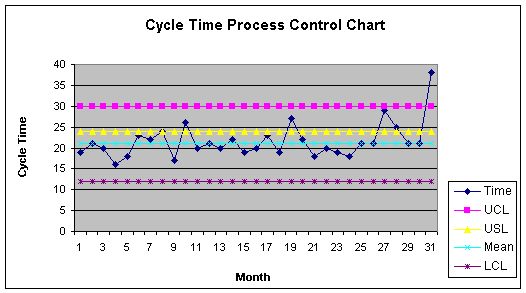Note that the 31st observation is above the UCL and would justify investigation as a signal that the process has gone out of control. Let's confirm this with finding the probability of getting an observation of 38 or greater, with a process mean of 21 and standard deviation of 3. First, the Z-Score computation:

Z-Score = (number - mean)/Std Dev = (38 - 21)/3 = 5.67

Now, find the probability using NORMSDIST function of Excel. The cell formula is:

=NORMDIST(5.67) = 0.99999999

Recall, this is the cumulative probability of observing a number LESS than 38. So to get the probability of observing a number greater than 38, take 1.0 - .99999999 = 0.00000001 or there is a 0% probability of observing a 38 if the mean is 21 and the standard deviation is 3 - that's why its called a signal or outlier.

Before leaving the process control chart, let's focus for a moment on the upper specification limit set usually by the boss or customer. The USL is 24. Way back at the beginning of this note set, Module 1.1, we indicated that there is no way we can please the boss with an upper specification limit of 24. Now you should be able to say why that is so. With a mean of 21 and a standard deviation of 3, we know that 24 is +1 standard deviations from the mean ( z- score = (24 - 21)/3 = + 1). The probability of getting an observation above 24 is found by first getting the probability of a number less than 24:

=NORMDIST(+1) = 0.84

This process is only capable of satisfying the customer 84% of the time - that is a measure of process capability. Now, to get the probability of finding a number above 24: 1.0 - 0.84 = 0.16. There is a 16% probability of getting an observation above 24 - that is why the customer will not be pleased. An upper specification limit one standard deviation above the mean is a 1 sigma process - GE's goal is to have SIX SIGMA processes - specification limits 6 standard deviations from the mean.

Descriptive Statistics
What if you were just starting out and collected 31 observations and had no prior knowledge of the mean, standard deviation, median, IQR or histogram. You would first generate the histogram, as shown in Worksheet 1.3.4 below.

Worksheet 1.3.4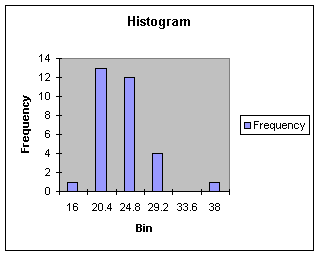By observation, we would say there is an outlier to the right of the range of the data, thus making a distribution that is skewed right. If we needed to report the center and spread of the skewed distribution we would use the....... that's right, the median and interquartile range. Here are the descriptive statistics:

Worksheet 1.3.5
 Time Mean 21.61 Standard Error 0.75 Median 21 Mode 21 Standard Deviation 4.19 Sample Variance 17.58 Kurtosis 7.04 Skewness 2.22 Range 22 Minimum 16 Maximum 38 Sum 670 Count 31 Confidence Level(95.0%) 1.54 1st Quartile = 19 3rd Quartile = 22.5 Interquartile Range = 3.5

Using this data, we see that the mean is now greater than the median, confirming the right skew. Note that the median remains 21, as with the original data set. The mean is larger since the outlier is pulling it to the right. Note also the standard deviation is 4.19 or 4.2 which is considerably inflated from the original data with its standard deviation of 3. Outliers always increase the standard deviation. To confirm that 38 is an outlier with this data, we standardize:

=STANDARDIZE(38,21.6,4.2) = 4.05

Thirty-eight is more than 3 standard deviations from the mean. In a real project, we would segregate the 38 from the rest of the data, investigate and make corrections, and redo the descriptive analysis without the outlier. This is precisely what you may have to do with your data in Project Assignment 1. Item 5 is to standardize your data, Item 6 is to remove outliers and re-compute the descriptive statistics. Data without outliers is likely to be symmetric, bell shaped if there was no bias in data collection. Inferential statistics for the mean of a distribution, which includes confidence intervals and tests of hypothesis, work best with symmetric bell shaped distributions. These subjects are covered next in Modules 1.4 and 1.5.

Optional Material on the Mean

This module focused on the arithmetic mean, the typical measure of central tendency, and the measure used in inferential statistics. There are two other means I should briefly mention: the weighted mean, and the geometric mean.

Weighted Mean
The weighted mean is a special case of the arithmetic mean used when there are several observations of the same value. Suppose you had cycle times of 21, 23, 19, 22, and 20, which gives an arithmetic mean of 21 days. But suppose further that cycle time 21 happened 20 times, 23 happened 5 times, 19 happened 50 times, 22 happened 10 times, and 20 happened 15 times. The mean cycle time weighted by frequency of occurrence, or simply the weighted mean is:

Weighted mean = sum of weights * observation / sum of weights
where weights are frequency of occurrence in this example

Weighted mean = (21*20 + 23*5 + 19*50 + 22*10 + 20*15) / 100 = 20

Geometric Mean
The geometric mean applies when we are interested in computing the mean for interest and growth rates, percentages, and ratios (Mason, 1999). Suppose you have a CD paying 10% interest in Year 1 (I wish!), and then 4% interest in Year 2. The arithmetic mean interest rate is

Mean interest rate, i = (0.10 + 0.04 ) / 2 = 0.07 or 7 %

However, to take into account the compounding effect, the geometric mean gives a more precise outcome:

Geometric mean = (1 + i) = nth root [ (1+i) * (1+i) ]
= square root [ (1+0.10) * (1+0.04) ] = 1.0696 or 6.958 %

Let's check this with \$95,000:

95,000 * 0.10 = 9500 (Year One)
(95000 + 9500) * 0.04 = 4180 (Year Two)
Total Interest = 9500 + 4180 = 13680

The geometric mean: 95000 * 0.6958 = 6610
(95000 + 6610) * 0.04 = 7070

Total Interest = 6610 + 7070 = 13680

The arithmetic mean: 95000 * 0.07 = 6650
(95000 + 6650) * 0.07 = 7115

Total Interest = 6650 + 7115 = 13765

The geometric mean gives the truer figure in recognition of the compounding effect. In general: geometric mean = nth root [x1x2...xn] where all x values must be positive.

A second application of the geometric mean is to find the percent increase, or growth rate, over time. Suppose the University gave the College of Business the following head count growth targets: 182 MBA students in 2001 and 344 MBA students in 2005. The geometric mean percent increase over time is:

= (n-1) root of [value at end of period/value at beginning of period] - 1
= (5 years - 1) or 4th root of [344 / 182 ] - 1
= .1725 or 17.25%

Reference:

Anderson, D., Sweeney, D., & Williams, T. (2001). Contemporary Business Statistics with Microsoft Excel. Cincinnati, OH: South-Western, Chapter 3 (except Section 3.5) and Chapter 6 (Section 6.2).

Mason, R., Lind, D. & Marchal, W. (1999). Statistical Techniques in Business and Economics (10th. ed.). Boston: Irwin McGraw Hill, Chapter 2.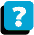About the Course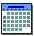Module Schedule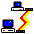WebBoard